## Zig Zag Matrix In sas

Hi Friends,

I have a query, I need the code for the below output, Could you please me on this. Its the Zig Zag concept I need to implement in SAS.

 Order Day1 Day2 Day3 Day4 Day5 Day6 Day7 Day8 Day9 Day10 1 1 3 6 10 14 18 ? ? ? ? 2 2 5 9 13 17 21 ? ? ? ? 3 4 8 12 16 20 23 ? ? ? ? 4 7 11 15 19 22 24 ? ? ? ?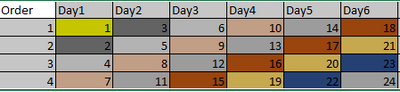The above table for 4*6 table -- I need to create a table automatically n rows and n columns with the same logic.

1 ACCEPTED SOLUTION

Accepted Solutions

## Re: Zig Zag Matrix In sas

Here is method using a temporary 2-D matrix.

``````%let rows=4;
%let cols=6 ;

data want;
array x[&rows,&cols] _temporary_ ;
array out day1-day&cols ;

do diag=1 to &rows+&cols-1;
row=min(&rows,diag);
col=1 + max(0,diag-&rows);
do while(1<=row and col<=&cols);
counter+1;
x[row,col]=counter;
col+1;
row+-1;
end;
end;

do row=1 to &rows;
do col=1 to &cols;
out[col] = x[row,col];
end;
output;
end;
drop diag row col counter ;
run;

proc print;
run;``````
```Obs    day1    day2    day3    day4    day5    day6

1       1       3       6      10      14      18
2       2       5       9      13      17      21
3       4       8      12      16      20      23
4       7      11      15      19      22      24
```
14 REPLIES 14

## Re: Zig Zag Matrix In sas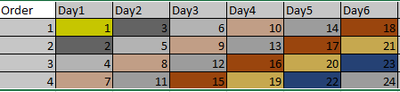The above table for 4*6 table -- I need to create a table automatically n rows and n columns with the same logic.

## Re: Zig Zag Matrix In sas

There are at least two possible approaches:

1. In a loop from 1 to m*n (where m is the number of rows and n the number of columns of the matrix) compute the (row, column) coordinates (i, j) of the matrix element where the value of the index variable will occur. Then store this value in a lookup table (e.g., two-dimensional array or hash object). Once the lookup table has been populated, write it to a dataset.
2. In two nested loops from 1 to m and 1 to n compute the value that is to occur at the position defined by the values, say, i, j of the two index variables. Then those computed values can be written row by row to a dataset.

Maybe approach #1 would be more elegant, but here is an implementation of approach #2:

``````%macro zigzag(m,n,out=want);
data &out(rename=(i=Order) drop=j k);
do i=1 to &m;
array Day[&n];
do j=1 to &n;
k=i+j-2;
Day[j]=(k*k+k
-(k>&m)*(k-&m)*(k-&m+1)
-(k>&n)*(k-&n)*(k-&n+1))/2 + min(j,&m-i+1);
end;
output;
end;
run;
%mend zigzag;

%zigzag(4,6)

proc print data=want noobs;
run;``````

You may want to run a whole series of test cases, which is the purpose of macro testzz below:

``````%macro testzz(maxrows,maxcols,out=want);
%local m n;
%do m=1 %to &maxrows;
%do n=1 %to &maxcols;
%zigzag(&m,&n,out=&out)
title "m=&m n=&n";
proc print data=&out noobs;
run;
%end;
%end;
title;
%mend testzz;

%testzz(6,6)``````

(Edit: minor simplifications)

## Re: Zig Zag Matrix In sas

Here is method using a temporary 2-D matrix.

``````%let rows=4;
%let cols=6 ;

data want;
array x[&rows,&cols] _temporary_ ;
array out day1-day&cols ;

do diag=1 to &rows+&cols-1;
row=min(&rows,diag);
col=1 + max(0,diag-&rows);
do while(1<=row and col<=&cols);
counter+1;
x[row,col]=counter;
col+1;
row+-1;
end;
end;

do row=1 to &rows;
do col=1 to &cols;
out[col] = x[row,col];
end;
output;
end;
drop diag row col counter ;
run;

proc print;
run;``````
```Obs    day1    day2    day3    day4    day5    day6

1       1       3       6      10      14      18
2       2       5       9      13      17      21
3       4       8      12      16      20      23
4       7      11      15      19      22      24
```

## Re: Zig Zag Matrix In sas

Thank you very much for your help with this, it's working fine.   I really impressed with your coding skills.Ksharp
Super User

## Re: Zig Zag Matrix In sas

It is easy for IML.

``````%let nrow=4;
%let ncol=6;

proc iml;
count=0;
max=&nrow <> &ncol;
x=j(max,max,0);

do n=2 to 2#max;
temp=loc(row(x)+col(x)=n);
idx=idx//temp[ncol(temp):1];
end;

dim=nrow(x)||ncol(x);
s=ndx2sub(dim,idx);
do i=1 to nrow(s);
if s[i,1]<=&nrow & s[i,2]<=&ncol then do;
count=count+1;
full=full//(count||s[i,]);
end;
end;
want=full(full);

create want from want[c=("day1":"day&ncol")];
append from want;
close;
quit;

proc print;run;``````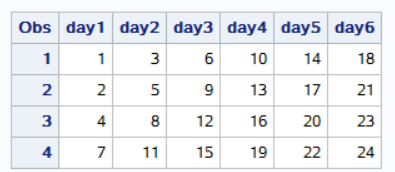## Re: Zig Zag Matrix In sas

Okay I will try to learn IML, Thanks for your response

## Re: Zig Zag Matrix In sas

You've seen a row-by-row solution with direct calculation of each element of a given row.  And a serpentine solution that winds through the matrix.

This is a row by row solution in which only row 1 is "directly" calculated. Every subsequent row is calculated from the prior row   (i.e.   X{i,j}=X{i-1,j+1}-1).

Row 1 has day1=1,     day2=day1+2, day3=day2+3,  etc. up to a maximum increment of the number of  rows.    And the last &norders increments (for the "extra" array elements below) will be   4,3,2,1  (for &norders=4).

My first program failed to properly set up the values for order 1 (thanks @Ksharp ).  Here's the corrected version:

``````%let norders=4;
%let ncols=10;
%let array_length=%eval(&ncols+&norders);

data want (drop=_:);
order=1;
array days {&array_length} day1-day&ncols  _dummy1-_dummy&norders (1);

do order=1 to &norders;
do _d=ifn(order=1,2,1) to &array_length-order+1;
_d2=&array_length - _d + 1 ;
if order=1 then days{_d}= days{_d-1}+min(_d,_d2,&norders);
else days{_d}=days{_d+1}-1;
end;
output;
end;

run;``````

The array is given the length of  NORDERS+NCOLS, (not just NCOLS), which is fully populated for order1.  This allows the diagonal copying to provide valid values for the lower right segment of the matrix.

Also the first element of array DAYS is initialized to a 1, which make programming the do loop iteration over days easier (do _d=2 to &array_length for order=1,   but _d=1 to &array_length-order+1 for other orders).

The _d2 variable is for counting down from the upper bound of the array.

--------------------------
The hash OUTPUT method will overwrite a SAS data set, but not append. That can be costly. Consider voting for Add a HASH object method which would append a hash object to an existing SAS data set

Would enabling PROC SORT to simultaneously output multiple datasets be useful? Then vote for
Allow PROC SORT to output multiple datasets

--------------------------

## Re: Zig Zag Matrix In sas

I like that idea.

Basically the increment needed for the first row's value in a 4 row by 6 col solution is:

```COL:   1  2  3  4  5  6  7  8  9 10
Value: 1  3  6 10 14 18 22 25 27 28
Diff:  1  2  3  4  4  4  4  3  2  1```

So the amount to increment increases by one up to the number of ROWS and stays constant until reaching the number of columns, and then the increment decreases by one each time.

``````%let rows=4 ;
%let cols=6 ;
data want ;
do row=1 to &rows;
array x day1-day&cols d1-d&rows ;
if row=1 then do increment=1 to &cols,&rows to 1 by -1 ;
col+1;
next+min(&rows,increment);
x[col]=next;
end;
else do col=1 to dim(x)-1;
x[col]=x[col+1]-1;
end;
output;
end;
drop next col increment d1-d&rows;
run;``````

Note the advantage of my first solution using the 2-D temporary array is that it is easy to adapt to fill the "matrix" with values from a dataset instead of the just the sequence 1,2,3,...Ksharp
Super User

## Re: Zig Zag Matrix In sas

I found another version of Zig Zag Matrix in internet.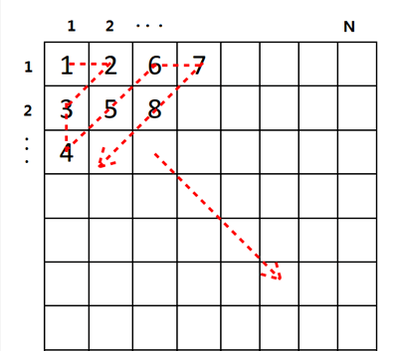How do you get it ?

I post IML code firstly here .

``````%let nrow=4 ;
%let ncol=6;

proc iml;
max=&nrow <> &ncol;
j=j(max,max,0);
do n=2 to 2#max;
temp=loc(row(j)+col(j)=n);
if mod(n-1,2)=1 then idx=idx||t(temp[ncol(temp):1]);
else idx=idx||temp;
end;
dim=max||max;
sub=ndx2sub(dim,idx) ;
count=0;
do i=1 to nrow(sub);
if sub[i,1]<=&nrow & sub[i,2]<=&ncol then do;
count=count+1;
full=full//(count||sub[i,]);
end;
end;
want=full(full);
create want from want[c=("date1":"date&ncol")];
append from want;
close;
quit;

proc print;run;``````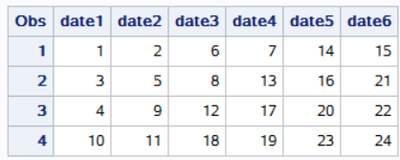## Re: Zig Zag Matrix In sas

So that one is alternating the order that the diagonals are traversed instead of always going in the same direction.

``````%let rows=4;
%let cols=6;

data want;
array x[&rows,&cols] _temporary_ ;
array out day1-day&cols ;
do diag=1 to &rows+&cols-1;
direction=-1+2*mod(diag,2);
if direction=1 then do;
row=min(diag,&rows);
col=1 + max(0,diag-&rows);
end;
else do;
col=min(diag,&cols) ;
row=1 + max(0,diag-&cols);
end;
do while(1<=row<=&rows and 1<=col<=&cols);
counter+1;
x[row,col]=counter;
col+direction;
row+-direction;
end;
end;

do row=1 to &rows;
do col=1 to &cols;
out[col] = x[row,col];
end;
output;
end;
drop diag row col direction counter ;
run;``````

To modify the above code to replicate the original ZigZag order just force DIRECTION to always be one instead of alternating between 1 and -1.Ksharp
Super User

## Re: Zig Zag Matrix In sas

Tom,
Cool. It is great honor for pfizer to have you vice-president .Ksharp
Super User

## Re: Zig Zag Matrix In sas

mkeintz,

When I set the following:

%let norders=40;
%let ncols=2;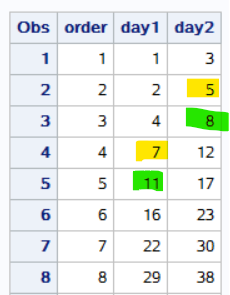Ksharp
Super User

## Re: Zig Zag Matrix In sas

I still get wrong result when I set this :

%let norders=40;
%let ncols=2;
%let array_length=%eval(&ncols+&norders);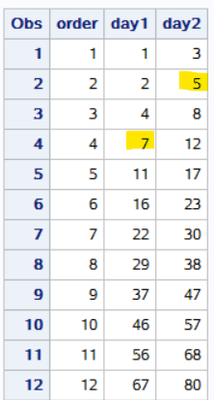Discussion stats
• 14 replies
• 2346 views
• 14 likes
• 5 in conversation